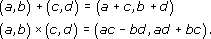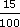Look at the shapes below. The symmetry of the shape on the left and its relationship to the shape on the right can be thought of in two ways:

• Fold the left-hand shape along the central line. Then one side lies exactly on top of the other, and gives the shape on the right.

• Imagine a mirror placed along the central dotted line. The reflection in the mirror gives the other half of the shape.

Author(s): The Open University

Symmetry is a feature that has been used in the design of objects and patterns in many cultures throughout recorded history. From Greek vases and medieval windows to Victorian tiles and Native American decorations, symmetry has been seen as a way of achieving balance and beauty.

All circles are the same shape – they can only have different sizes.

In a circle, all the points are the same distance from a point called the centre. The centre is often labelled with the letter O.Author(s): The Open University

You can use the fact that the sum of angles at a point is 360° to draw a pie chart.

## Example 4

Over a five-year period a mathematics tutor found that 16 of her students gained distinctions, 32 gained pass grades and 12 failed to complete the course. Draw a pie chart to re
Author(s): The Open University## Study another free course

There are more than 800 courses on OpenLearn for you to
Author(s): The Open University

In this section we have seen that the complex number system is the set R × R together with the operations + and × defined byFrom this, one can justify the performance of ordinary algebraic operations on expressions of the form
Author(s): The Open University

In Section 5 we show how functions may be used to sketch curves in the plane, even when these curves are not necessarily the graphs of functions.

Click the link below to open Section 5 (8 pages, 151KB).

Section 5

Author(s): The Open University## Study another free course

There are more than 800 courses on OpenLearn for you t
Author(s): The Open University

Discount can be calculated in the same way as an increase by a percentage. For example, £8 with 15% discount means you actually pay

£8 less (15% of £8)

15% of 8 =× 8 =
Author(s): The Open University

## Activity 20

Convert each of the following to percentages. Round off the percentages to whole numbers.

• (a)

• (i) 0.8

• (ii) 0.
Author(s): The Open University

## Activity 17

Express each of the following percentages as fractions:

• (a) 40%

• (b) 8%

• (c) 70%

• (d)
Author(s): The Open University

## Activity 14

A piece of computer software is to be developed by a team of programmers. It is estimated that a team of four people would take a year. Which of the following times is the length of time taken by three program
Author(s): The Open University

## Activity 5

Convert each of the following fraction ratios to decimal ratios.

• (a) [Image_Link]https://www.open.edu/op
Author(s):
The Open University

Time conversions are also ratios. The ratio of time measured in minutes to time measured in seconds is one to sixty (1:60), as there are sixty seconds in a minute.

## Example 2

Adam's grandfather ran a mile in
Author(s): The Open University

To make short crust pastry, one recipe book says ‘use one part of fat to two parts of flour’; another recipe says ‘use fat and flour in the ratio of one to two’; and yet another says ‘use half as much fat as flour’. These are different ways of expressing the same ratio. Ratios are often expressed as fractions. So in this case:

[Image_Link]https://www.open.edu/openlearn/ocw/pluginfile.php/
Author(s): The Open University

The content acknowledged below is Proprietary (see and conditions made available under a Creative Commons Attribution-NonCommercial-ShareAlike 4.0 Licence) and used under licence.

Course image: rod
Author(s): The Open University

Ahmed, A. (1987) Better Mathematics, London, HMSO.
DfEE (2001) Key Stage 3 National Strategy: Framework for Teaching Mathematics: Years 7, 8 and 9, London, DfEE.
NCTM (1989) Curriculum and Evaluation Standards for School Mathematics Reston VA, National Council of Teachers of Mathematics.

Author(s): The Open University

In this course you have been introduced to the difference between mathematical content and processes. You have worked on the do–talk–record (DTR) framework for learning mathematics.

Author(s): The Open University

The do–talk–record triad (DTR) is a description of what is likely to take place in collaborative mathematics classrooms. It is concerned with observable events, and with the learner rather than the teacher, though many teaching insights flow from it. Although the order of the triad suggests that it should be followed in a particular sequence, this is not necessarily the case. Sometimes talking comes before doing or recording before talking. It also takes time for a learner to move
Author(s): The Open University

You will come to this course with many memories of mathematics, both as a teacher and a learner. It may help if you start by recalling memories of learning mathematics and making a record of them in your notebook.

When you work on a task, get into the habit of having your notebook to hand to record your thinking. Use the notebook in any way that helps you to think about the work you have done. Some people find it helpful to divide a page into two columns using the left-hand side to reco
Author(s): The Open University